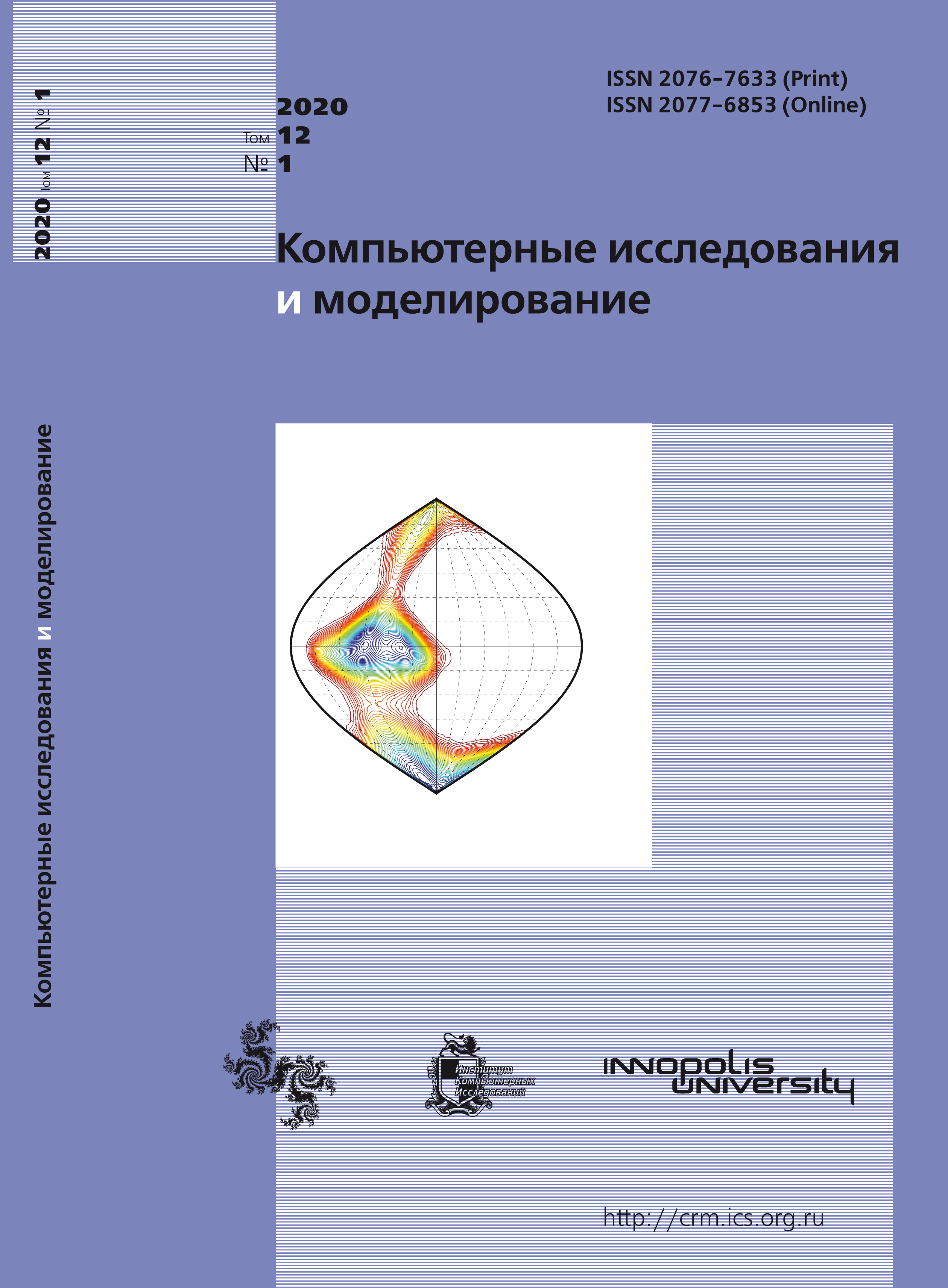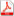Issue 1, 2020 Vol. 12

# All issues

Singular solutions of the multidimensional differential Clairaut-type equations in partial derivatives with trigonometric functionspdf (298K)

We study the class of first order differential equations in partial derivatives of the Clairaut-type, which are a multidimensional generalization of the ordinary differential Clairaut equation to the case when the unknown function depends on many variables. It is known that the general solution of the Clairaut-type partial differential equation is a family of integral (hyper-) planes. In addition to the general solution, there can be particular solutions, and in some cases a special (singular) solution can be found.

The aim of the paper is to find a singular solution of the Clairaut-type equation in partial derivatives of the first order with a special right-hand side. In the paper, we formulate a criterion for the existence of a special solution of a differential equation of Clairaut type in partial derivatives for the case, when the function of the derivatives is a function of a linear combination of partial derivatives of unknown function. We obtain the singular solution for this type of differential equations with trigonometric functions of a linear combination of $n$-independent variables with arbitrary coefficients. It is shown that the task of finding a special solution is reduced to solving a system of transcendental equations containing initial trigonometric functions. The article describes the procedure for evaluation of a singular solution of Clairaut-type equation; the main idea is to find not partial derivatives of the unknown function, as functions of independent variables, but linear combinations of partial derivatives with some coefficients. This method can be used to find special solutions of Clairaut-type equations, for which this structure is preserved.

The work is organized as follows. The Introduction contains a brief review of some modern results related to the topic of the study of Clairaut-type equations. The Second part is the main one and it includes a formulation of the main task of the work and describes a method of evaluation of singular solutions for the Clairaut-type equations in partial derivatives with a special right-hand side. The main result of the work is to find singular solutions of the Clairaut-type equations containing trigonometric functions. These solutions are given in the main part of the work as an illustrating example for the method described earlier. In Conclusion, we formulate the results of the work and describe future directions of the research.

Keywords: partial differential equations, Clairaut-type differential equations, singular solutions, trigonometric functions
Citation in English: Ryskina L.L. Singular solutions of the multidimensional differential Clairaut-type equations in partial derivatives with trigonometric functions // Computer Research and Modeling, 2020, vol. 12, no. 1, pp. 33-42
Citation in English: Ryskina L.L. Singular solutions of the multidimensional differential Clairaut-type equations in partial derivatives with trigonometric functions // Computer Research and Modeling, 2020, vol. 12, no. 1, pp. 33-42
DOI: 10.20537/2076-7633-2020-12-1-33-42

Full-text version of the journal is also available on the web site of the scientific electronic library eLIBRARY.RU

The journal is included in the Russian Science Citation Index

The journal is included in the List of Russian peer-reviewed journals publishing the main research results of PhD and doctoral dissertations.

International Interdisciplinary Conference "Mathematics. Computing. Education"

The journal is included in the RSCI

Indexed in Scopus